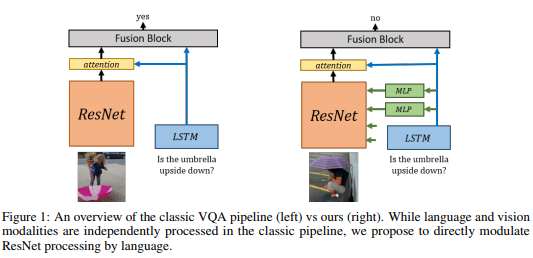Conditional Batch Normalization 的概念来源于这篇文章：Modulating early visual processing by language 。后来又先后被用在 cGANs With Projection DiscriminatorSelf-Attention Generative Adversarial Networks 。本文将首先简略介绍 Modulating early visual processing by language ，接着结合 Self-Attention GANs 的 pytorch 代码，详细分析 categorical conditional Batch Normalization 的具体实现。

## 太长不看版## Modulating early visual processing by languagehttps://github.com/ap229997/Conditional-Batch-Norm/blob/master/model/cbn.pygithub.com

## Categorical Conditional Batch Normalization

Modulating early visual processing by language 一文中，由于使用了预训练的 ResNet，不敢对预训练网络 BN 层的参数做大修改，因此 MLP 的输出为 BN 层参数的增量，而不是直接输出新的 BN 层参数。conditional GANs 没有用到预训练网络，因此没有了历史包袱，直接用图片的 categorical 信息，预测新的class ConditionalBatchNorm2d(nn.BatchNorm2d):

"""Conditional Batch Normalization"""

def __init__(self, num_features, eps=1e-05, momentum=0.1,
affine=False, track_running_stats=True):
super(ConditionalBatchNorm2d, self).__init__(
num_features, eps, momentum, affine, track_running_stats
)

def forward(self, input, weight, bias, **kwargs):
self._check_input_dim(input)

exponential_average_factor = 0.0

if self.training and self.track_running_stats:
self.num_batches_tracked += 1
if self.momentum is None:  # use cumulative moving average
exponential_average_factor = 1.0 / self.num_batches_tracked.item()
else:  # use exponential moving average
exponential_average_factor = self.momentum

output = F.batch_norm(input, self.running_mean, self.running_var,
self.weight, self.bias,
self.training or not self.track_running_stats,
exponential_average_factor, self.eps)
if weight.dim() == 1:
weight = weight.unsqueeze(0)
if bias.dim() == 1:
bias = bias.unsqueeze(0)
size = output.size()
weight = weight.unsqueeze(-1).unsqueeze(-1).expand(size)
bias = bias.unsqueeze(-1).unsqueeze(-1).expand(size)
return weight * output + bias 

        if weight.dim() == 1:
weight = weight.unsqueeze(0)
if bias.dim() == 1:
bias = bias.unsqueeze(0)
size = output.size()
weight = weight.unsqueeze(-1).unsqueeze(-1).expand(size)
bias = bias.unsqueeze(-1).unsqueeze(-1).expand(size)
return weight * output + bias 

class CategoricalConditionalBatchNorm2d(ConditionalBatchNorm2d):

def __init__(self, num_classes, num_features, eps=1e-5, momentum=0.1,
affine=False, track_running_stats=True):
super(CategoricalConditionalBatchNorm2d, self).__init__(
num_features, eps, momentum, affine, track_running_stats
)
self.weights = nn.Embedding(num_classes, num_features)
self.biases = nn.Embedding(num_classes, num_features)

self._initialize()

def _initialize(self):
init.ones_(self.weights.weight.data)
init.zeros_(self.biases.weight.data)

def forward(self, input, c, **kwargs):
weight = self.weights(c)
bias = self.biases(c)

return super(CategoricalConditionalBatchNorm2d, self).forward(
input, weight, bias)

        self.weights = nn.Embedding(num_classes, num_features)
self.biases = nn.Embedding(num_classes, num_features)

nn.Embedding层的作用是，把图片的 label 转换成 dense 向量，而不像 one-hot-encoding，只能把 label 转换成稀疏向量。nn.Embedding的第一个参数表示总共有多少个类，第二个参数表示每个 label 映射成多少维的向量。这个网络层的好处是，可以任意指定 label vector 的 dimension，它的本质是一个 num_classesnum_feature列的矩阵，这个矩阵的参数随着网络的训练不断更新。前向传播时，label 是几就取第几行的向量出来，用以表示这个 label。其实这个 Embedding 相当于把 one-hot encoding 输入一个 bias 为 0 的 linear layer。

    def forward(self, input, c, **kwargs):
weight = self.weights(c)
bias = self.biases(c)

return super(CategoricalConditionalBatchNorm2d, self).forward(
input, weight, bias)

1. 原始论文中，基于的条件是 LSTM 提取的自然语言信息，而在这里的条件是图片的类别信息。
2. 原始论文中把 LSTM 提取的信息通过两个小神经网络（每个小网络都是 2 层 linear layers，中间夹着一个 ReLU），映射为。这里的 categorical 信息，直接通过 embedding layer 映射到向量，由于 embedding layer 本身相当于一个 one-hot-encoding+linear layer的组合，因此，这里实际上是把原文中的 linear+ReLU+ linear 小网络变成了一层 linear layer，原因大概是，比起自然语言的信息， categorical 信息太稀疏了，没有映射两遍的必要。
3. 原始论文中，MLP 的输出为的增量：。原因上文也提到过，是因为不能轻易改变预训练的 ResNet 中 BN 的参数，只能针对具体的 condition ，在预训练 BN 的参数上做小改。而 Categorical Conditional Batch Normalization 没有历史包袱，可以直接预测4. 原始论文中，输出的代入公式(2) 和 BN 参数整合到一起，做一遍映射就好了。而这里，是在原始的 BatchNorm2d映射之后，又做了一遍映射，我猜主要是为了实现起来比较方便，毕竟求 moving average 设置 momentum 这些杂活，实现起来比较麻烦，不如直接借用 pytorch 在 BatchNorm2d里的官方实现。